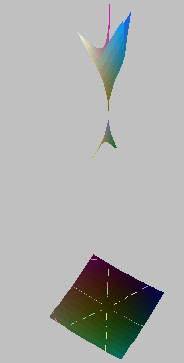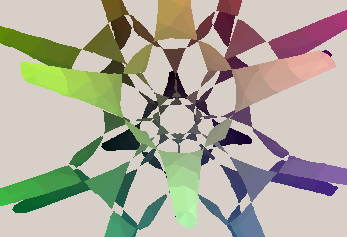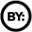# Release Notes

## Version 1/sqrt(tau) = 0.78615138, 6 June 2003

A major update of the asurf program. It has now can give almost perfect results for most types of singularities. Specifically

• It can read a data file with the known positions of singularities. Used with Oliver Labs' program which incorperates Singular (for algerbraic computation) and Surf for raytracing images.
• More work has gone into the correct treatment of faces and regions containing singularities. Several new conditions are now tested to try to deduce the geometry, especially cross-caps, cuspidal edges and triple points where second derivative info is now used.
• The routine for merging facets has been rewritten. Some post processing is caried out to remove unwanted facets.
• Several major speedups obtained by changing the order of computation. (3 routines take 75% of the time).
• A new web page with a pictorial index of all the surfaces added. Split in Asurf and Pro versions.
• Theres now a few easter eggs in the syntax of equations:
```# The following selects a specific box at the specified depth,
# only the component inside that box is drawn.
asurf_selx=8;asurf_sely=8;asurf_selz=8;asurf_denom=16;
# This line causes the curves df/dx=0, df/dy=0, df/dz=0 to be drawn.
asurf_degen=0;
```

## Version (1+sqrt(5))/2-1 = 1/tau = tau-1 = 0.61803399 May Day 2003Interim release hence the irational version number.

### Major new features:

The mapping, intersect, and icurve programs have not been adapted to run under JavaView. These allow such things as Parabolic lines, Ridges, Symmetry Sets and focal surfaces to be calculated.

The algebraic surface program has also been improved to make a better job around singular points.

### Major missing features: (yet to come)

I'd like to add better interactive control for the parameters in the equations.
Still some bugs in teselating algebraic surfaces and the boundaries are still not correct.
The communication protocol is still not standardised, some are using CGI type encoding, others are using an XML format which all programs are migrating to.
The server programs could all do with a clean up and actually get round to freeing some memory!
No documentation apart from this for new programs.
The icuve program currently only produces integral curves, computing vector fields and wavefronts yet to come.

### A few more specifics on the changes

The mapping, intersect and icurve programs all perform some operation on another geometry.
• The mapping program applies some mapping f: R^3 -> R^3 to the geometry.
• The intersect program will find the point of a geometry where f(x,y,z) = 0 (or > 0 or < 0) for some real valued function f.
• The icurve program uses the points of the input geometry as base points for calculating the integral curves for somevector field.
Furthermore each program can read part of the defining equation for the function from another geometry. For example the it is posible to write a generic project onto surface mapping, which can take parameterised surface and any set of points in parameter sace and project them onto the surface. i.e.
Generic project onto surface mapping + definition of surface = mapping to project onto the surface
This feature makes it easy to implement many standard local differential geometry functions:
Parabolic Lines, Ridge, Principal Directions, Focal Surfaces, Symmetry Sets etc.

There have been two major improvments to the algebraic surface program:
1. The routine to produce the polygons once all the points on the surface have been found has been completely changed. It now calculates facets for the surface at the bottom (smallest size = fine) level of recursion, these facets are then joined together higher up the recursion tree to produced the polygons used in the final surface (ie. at corse resolution). This is the technically correct way to do it rather than the add-hoc algorithms used previously. Thankfully this did not entail the expected increase in compilation time.
2. Convergence routines are now used to find the singular points, and the turning points. This means that far more acurate positions of singular points are found. (Warning still not converging to normal turning points, so surfaces are a little thiner than they should be).As well as these a bit more mathematics has been used to work out if a singularity should lie in a box. Second derivatives are now examined in more detail. In particular the determinant of the second derivative matrix is now examined. The sign of this gives the diference between two lines crossing on a face (x^2-y^2=0) or an isolated point on the face (x^2+y^2=0) and similar fun in 3D. This has helped reduce the number of false positive singurlarity points found.

The upshot of all these improvments is that singularities are much sharper as the picture of Sarti's octic with 144 double points shows.

### To Do

Long term stuff to do.

• Continue migration to pure java. At the moment the limiting feature is getting a sosphisticated equation parser in java. JEP looks good, but does not handle multiple equations, symbolic differentation, vector operations. Once thats done all the programs apart from the algebraic surface program can be implemented easily in pure java which will make things wiz, (communicating between server and client is the slowest bit).
• Other improments include standadising the comunication standard to use xml format for definitions in the end it will be something like:
```<definition name="Kummer surface" type="asurf">
(3-v^2) ( x^2+y^2 + z^2 - v^2)^2 - (3 v^2 - 1) p q r s = 0;
p = 1 - z - x rt2;
q = 1 - z + x rt2;
r = 1 + z + y rt2;
s = 1 + z - y rt2;
rt2 = sqrt(2);
<variable name="x" min="-1" max="1">
<parameter name="v" value="1.1">
<option name="course" value="8">
....
</definition>
```
This will be the format definition will be saved in and used to communicate between client and server.
• Other minor interface improvments in the pipeling include: being able to specify which display the results appear in, (so parameter space could be viewed in one window). A limited scripting capability to automate repeated operation and interactive changing of parameter values.
• Asurf: Improve the treatment of singularities, esp 2nd derivatives for self intersections, cross caps, cuspidal edges etc.
• Asurf: Maybe use Sturm sequences for tricky edges.
• Asurf: Post-process output to fill in the few remaining gaps.
• Work on a generalised n-equations in m-dimensions algorithms, possibly using data from Oliver Labs using Singular.This work is licensed under a Creative Commons License. (You can copy, distribute and display this works but: Attribution is required, its for Non-Commercial purposes, and it's Share Alike (GNUish/copyleft) i.e. has an identical license.) It would also be nice if you let me know (rich@singsurf.org) if you link to, redistribute, make a derived work or do anything groovy with this information.